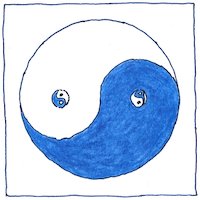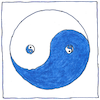# 1679 Binary number

## The book of science

Tom Sharp

 Gottfried Wilhelm Leibniz mathematics## Binary number

• Sumerian numbers
• were sexagesimal—base 60.
• Mayan, Aztec, Aruban numbers
• were vigesimal—base 20.
• Egyptian numbers
• were decimal—base 10.
• Yuki and Pamean numbers
• were octal—base 8.
• Numbers in a computer
• are binary—base 2—
• invented by G. W. Leibniz
• in 1679.

## Yin & yang

• Leibniz saw binary numbers
• in the hexagrams of the I Ching.
• Each line of a hexagram
• is either yin or yang.
• With six lines per hexagram
• you get sixth-four hexagrams.
• Leibniz saw in them an affirmation
• of the One and Nothing.

## Location arithmetic

• In 1617 John Napier described
• a non-positional notation
• for binary numbers.
• Instead of two symbols, 0 and 1,
• in Napier’s notation each letter
• of the alphabet represents a power of 2.
• The letter A represents
• 2 to the power of 0, equal to 1.
• The letter B represents
• 2 to the power of 1, equal to 2.
• The letter C represents
• 2 to the power of 2, equal to 4.
• The letter D represents
• 2 to the power of 3, equal to 8.
• To make a number,
• Napier combined letters in any order
• so that DAB, for example equals 11.
• You can replace each letter
• with two of the previous,
• and each doubled letter with the next.
• Addition is concatenation.
• Subtraction is removal.
• Multiplication by 2
• is replacing with the next.
• Division by 2
• is replacing with the previous.
• All odd numbers contain one A
• or an odd number of A letters.
• *
• In addition to the alphabetic symbols
• for non-positional powers of two,
• Napier proposed an alternative physical device,
• a grid of squares whose coordinates were powers of two.
• Napier’s grid, like other counting tables at the time,
• was similar in principle to the Chinese abacus.
• You could lay out tally markers or jetons
• represent numeric values, add, subtract,
• multiply, divide, and find square roots.

## Cipher

• In 1605 Francis Bacon described a cipher
• for hiding a secret message
• based on two intermixed typefaces
• that foreshadowed binary code.
• I learned as a programmer to recognize
• sequences of hexadecimal characters
• in memory dumps to debug my work.
• A dump is a cipher but not of a secret.
• To discover what went wrong
• I would check values in the registers
• bearing addresses of values in memory
• and try to reconcile these with what I expected.

The Sexagesimal numeral system gives us our hours, minutes, and seconds. Yuki octal numbers came about from counting, instead of the fingers themselves, the spaces between the fingers, perhaps because the spaces resembled containers better. There is nothing inherently advantageous about decimal numbers. Computer systems use binary (because a switch may be only on or off), and computer programmers use octal and hexadecimal as logical groupings for binary code, which work because 8 and 16 are powers of 2.

See also in The book of science: